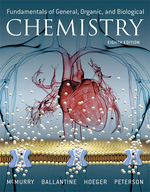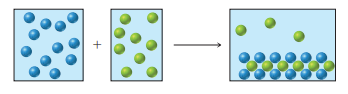×
Get Full Access to Fundamentals Of General, Organic, And Biological Chemistry (Mastering Chemistry) - 8 Edition - Chapter 5 - Problem 5.20
Get Full Access to Fundamentals Of General, Organic, And Biological Chemistry (Mastering Chemistry) - 8 Edition - Chapter 5 - Problem 5.20

×ISBN: 9780134015187 2044

## Solution for problem 5.20 Chapter 5

Fundamentals of General, Organic, and Biological Chemistry (Mastering Chemistry) | 8th Edition

• Textbook Solutions
• 2901 Step-by-step solutions solved by professors and subject experts
• Get 24/7 help from StudySoup virtual teaching assistantsFundamentals of General, Organic, and Biological Chemistry (Mastering Chemistry) | 8th Edition

4 5 1 376 Reviews
13
0
Problem 5.20

An aqueous solution of a cation (represented as blue spheres in the diagram) is allowed to mix with a solution of an anion (represented as green spheres) and the following result is obtained:Which combinations of cation and anion, chosen from the following lists, are compatible with the observed results? Explain.

Cations: $$\mathrm{Na}^{+}, \mathrm{Ca}^{2+}, \mathrm{Ag}^{+}, \mathrm{Ni}^{2+}$$

Anions: $$\mathrm{Cl}^{-}, \mathrm{CO}_{3}^{2-}, \mathrm{CrO}_{4}^{2-}, \mathrm{NO}_{3}^{-}$$

Text Transcription:

Na^2, Ca^2+, Ag+, Ni^2+

CO^-, CO_3^2-, CrO_4^2-, NO_3^-

Step-by-Step Solution:
Step 1 of 3

A- 3 i. Aluminum- Al ii. Argon-Ar iii. Arsenic- As B- 4 i. Barium- Ba ii. Beryllium- Be iii. Boron- B iv. Bromine- Br C- 7 i. Calcium- Ca ii. Carbon- C iii. Cesium- Cs iv. Chlorine- Cl v. Chromium- Cr vi. Cobalt- Co vii. Copper- Cu D- 0 E- 0 F- 2 i. Fluorine- F ii. Francium- Fr G- 3 i. Galium- Ga ii. Germanium- Ge iii. Gold- Au H- 2 i. Helium- He ii. Hydrogen- H I- 2 i. Iodine- I ii. Iron- Fe J- 0 K- 1 i. Krypton- Kr L- 2 i. Lead- Pb ii. Lithium- Li M- 3 i. Magnesium- Mg ii. Manganese- Mn iii. Mercury- Hg N- 3 i. Neon- Ne ii. Nickle- Ni iii. Nitrogen- N O- 1 i. Oxygen- O P- 2 i. Phosphorus- P ii. Potassium- K Q- 0 R- 2 i. Radium- Ra ii. Rubidium- Rb S- 7 i. Scandium- Sc ii. Selenium- Se iii. Silicon- Si iv. Silver- Ag v. Sodium- Na vi. Sulfur- S vii. Strontium- Sr T- 2 i. Tin- Sn ii. Titanium- Ti U- 0 V- 1 i. Vanadium- V W- 0 X- 0 Y- 0 Z- 1 i. Zinc- Zn

Step 2 of 3

Step 3 of 3

##### ISBN: 9780134015187

This full solution covers the following key subjects: . This expansive textbook survival guide covers 281 chapters, and 240 solutions. Fundamentals of General, Organic, and Biological Chemistry (Mastering Chemistry) was written by Aimee Notetaker and is associated to the ISBN: 9780134015187. This textbook survival guide was created for the textbook: Fundamentals of General, Organic, and Biological Chemistry (Mastering Chemistry), edition: 8. The full step-by-step solution to problem: 5.20 from chapter: 5 was answered by Aimee Notetaker, our top Chemistry solution expert on 04/25/22, 03:57PM. The answer to “?An aqueous solution of a cation (represented as blue spheres in the diagram) is allowed to mix with a solution of an anion (represented as green spheres) and the following result is obtained: Which combinations of cation and anion, chosen from the following lists, are compatible with the observed results? Explain. Cations: $$\mathrm{Na}^{+}, \mathrm{Ca}^{2+}, \mathrm{Ag}^{+}, \mathrm{Ni}^{2+}$$ Anions: $$\mathrm{Cl}^{-}, \mathrm{CO}_{3}^{2-}, \mathrm{CrO}_{4}^{2-}, \mathrm{NO}_{3}^{-}$$Text Transcription:Na^2, Ca^2+, Ag+, Ni^2+CO^-, CO_3^2-, CrO_4^2-, NO_3^-” is broken down into a number of easy to follow steps, and 68 words. Since the solution to 5.20 from 5 chapter was answered, more than 203 students have viewed the full step-by-step answer.

Unlock Textbook Solution